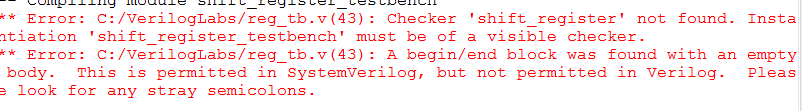Community
cancel
Showing results for
Did you mean:
Highlighted
Beginner
22 Views

Hey i wrote this code in Verilog.

This is the Code:

``````module shift_register(write,s_in,clk,d,s_out,p);

input write,clk;

input [3:0] d;
input [11:0] s_in;

output reg s_out;
output reg [3:0] p;

wire mux_1,mux_2,mux_3,mux_4,dff_1,dff_2,dff_3,dff_4; // 8 wires

mux2_1 mux1(.s(s_in),.d(d),.w(write),.z(mux_1)); // mux1

D_FlipFlop dff1(.d(mux_1),.clk(clock),.q(dff_1)); // DFlipFlop1
always@(posedge clk) begin
p<=dff_1;
end
mux2_1 mux2(.s(dff_1),.d(d),.w(write),.z(mux_2));// mux2

D_FlipFlop dff2(.d(mux_2),.clk(clock),.q(dff_2));  // DFlipFlop2
always@(posedge clk) begin
p<=dff_2;
end
mux2_1 mux3(.s(dff_2),.d(d),.w(write),.z(mux_3));// mux3

D_FlipFlop dff3(.d(mux_3),.clk(clock),.q(dff_3));  // DFlipFlop3
always@(posedge clk) begin
p<=dff_3;
end
mux2_1 mux4(.s(dff_3),.d(d),.w(write),.z(mux_4));// mux4

D_FlipFlop dff4(.d(mux_4),.clk(clock),.q(dff_4));  // DFlipFlop4
always@(posedge clk) begin
p<=dff_4;

s_out<=dff_4;
end
endmodule``````

and this is the Testbench:

``````module shift_register_testbench();
wire write,clk;

input [3:0] d;
input [11:0] s_in;

output reg s_out;
output reg [3:0] p;

initial
begin
d[3:0]  = 1'b1;
write = 1'b0;

s_in = 1'b1;
#10;
s_in = 1'b0;
#10;
s_in = 1'b0;
#10;
s_in = 1'b1;
#10;
s_in = 1'b0;
#10;
s_in = 1'b0;
write = 1'b1;
#10;
s_in = 1'b1;
write = 1'b0;
#10;
s_in = 1'b0;
#10;
s_in = 1'b1;
#10;
s_in = 1'b1;
#10;
s_in = 1'b1;
#10;
s_in = 1'b0;
#10;

shift_register SRTB(.write(write),.s_in(s_in[11:0]),.clk(clk),.d(d[3:0]),.s_out(s_out),.p(p[3:0]));

end
endmodule

``````

And when I`m try to run the testbench this problem appear:how can i fix it?THNX!

2 Replies
Highlighted
Valued Contributor III
7 Views

Where is your shift register HDL file located?  Is it in the same directory as the testbench?  I think the issue is ModelSim is simply not seeing the file.  Make sure you "Change Directory" in ModelSim to point to the location of the code.

#iwork4intel

Highlighted
Moderator
3 Views